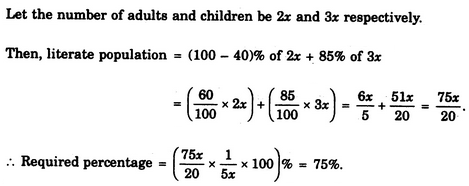Verbal Reasoning - Arithmetic Reasoning - Discussion

Discussion :: Arithmetic Reasoning - Section 1 (Q.No.30)

30.

In a city, 40% of the adults are illiterate while 85% of the children are literate. If the ratio of the adults to that of the children is 2 : 3, then what percent of the population is literate ?

 [A]. 20% [B]. 25% [C]. 50% [D]. 75%

Explanation:Harshwardhan said: (Dec 27, 2012) Men illiterate 40% = 60% literate Children= 85% literate 2:3 ratio 2*100:3*100 200:300 ==200+300=500 60% of 200: 85% of 300 120:255= 120+255=375 Now 375/500==75%

 Priya said: (Dec 13, 2013) Why you are subtracting 40% from 100 but not 85%. Will you clear it?

 Adp said: (Jan 17, 2014) We Have to find literate percent. For men it was given illiterate percent so we are subtracting from 100, but in case of children literate percents given so no need 2 subtract.

 Khadyoth said: (Feb 4, 2016) Why did you multiply 75x/20 with 100/5x? Why did you take 5x?

 Mathslover said: (Nov 7, 2016) Let A for adults and C for children. A : C :: 2 : 3. i.e. A : C = 40 : 60 (from 100 people). So iliterate adult 40% i.e. 40% of 40 people = 16 people (out of 100). So iliterate childs are 15% i.e. 15% of 60 people = 9 people (out of 100). So total iliterate people are 16 + 9 = 25 (from 100). Then literate people 100 - 25 = 75% (Percentage is because we take out of 100).

 Priya said: (Jul 23, 2017) @Khadyoth. We have divided the literate population fraction by the total population, i.e, 5x(2x+3x). And multiplied it with 100 to get the percentage. ((75x/20 ÷ 5x) * 100).

 Shivangi said: (Nov 26, 2017) Can't understand please anyone makes it clear.

 Niranva said: (Jul 22, 2018) Adult : Children. 2x=3x Literate=2 x (60%) + 3x(85%), =120x+255x, =375x. %= 375/500 = 75%.

 Talari Suresh said: (Nov 20, 2018) 100 - 85 = 15 % 100 - 40 = 60 % 15% + 60% = 75 %. Ans: 75%.

 Pankaj said: (Dec 31, 2018) Where from 1/ 5x came? Explain.

 Washington said: (Jan 22, 2019) How did you get that 1/5x? please explain.

 Nayana said: (Jun 3, 2019) The ratio of adults to children (total) = 2:3. So, adding these ratios up, we can conclude that out of 5 people, 2 of them are adults and 3 of them are children. So, adults = 2/5, children = 3/5. They have taken 1/5 as common, this is how 1/5 comes. hope it helps.